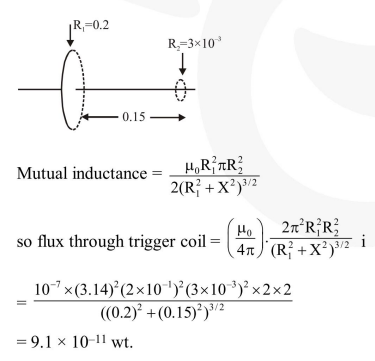Deepak Scored 45->99%ile with Bounce Back Crack Course. You can do it too!

# A circular loop of radius 0.3 cm lies parallel to a much bigger circular loop of radius 20 cm.

Question:

A circular loop of radius $0.3 \mathrm{~cm}$ lies parallel to a much bigger circular loop of radius $20 \mathrm{~cm}$. The centre of the small loop is on the axis of the bigger loop. The distance between their centres is 15 $\mathrm{cm}$. If a current of $2.0$ A flows through the smaller loop, then the flux linked with bigger loop is :-

1. $9.1 \times 10^{-11}$ weber

2. $6 \times 10^{-11}$ weber

3. $3.3 \times 10^{-11}$ weber

4. $6.6 \times 10^{-9}$ weber

Correct Option: 1

Solution: